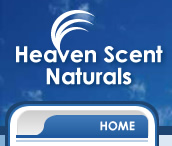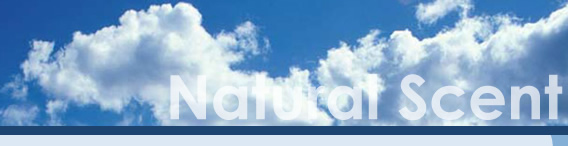Candle and Soap Making

### Candle Measurements

For many candle makers one of the most confusing things is measuring ingredients. In this feature I will try to take some of the mystery out of mixing wax formulas, and show you where to find on-line converters where applicable.

Formula Basics
The most accurate way to handle any candle making products is by weight. If you have a product that requires absolute repeatability, there is no way to avoid buying an accurate scale. Smaller batches of wax require more accurate scales since will be using small quantities of most ingredients. A word of advice here - shop around before buying a scale. When one of my scales died recently, I planned to buy a replacement triple beam. Upon looking around the web I found prices varying as much as 30% - for the same exact scale! After shopping a bit more, I realized that for a few dollars more I could get a digital scale which is a big time saver (although I doubt it will last as long as a triple beam scale).

Percentage Formulas
All percentage formulas add up to 100%. One percent is one onehundreth or 1/100. A sample percentage formula might look like this:
93% paraffin
1% Vybar 103
6% scent oil
If we wanted to make 100 pounds of this formula we would use 93 lbs of paraffin, 1 lb Vybar, and 6 lbs of scent. These same would hold true for 100 ounces or 100 grams.

Percentage formulas are easily scaled up or down. For example if we needed 10 pounds of this mix we would divide the 100 pound formula by 10 Giving us 9.3 lbs paraffin, 1/10 lb Vybar, and 6/10 lb of scent. To scale up we would multiply. For example if we needed 150 pounds of this formula, multiply all ingredients by 1.5

Summary
Putting this feature together has taken much longer than planned. Sometimes putting the things you do daily into words is much more difficult than actually doing them. Next week I will discuss proportional formulas, "weighing" wax without a scale, and more.